Contemporary Mathematics

# 12.3Comparing Graphs

Contemporary Mathematics12.3 Comparing Graphs

Figure 12.36 A flat map represents the surface of Earth in two dimensions. (credit: modification of work "World Map" by Scratchinghead/Wikimedia Commons, Public Domain)
Figure 12.37 A globe represents the surface of Earth in three dimensions. (credit: "Globe" by Wendy Cope/Flickr, CC BY 2.0)

### Learning Objectives

After completing this section, you should be able to:

1. Identify the characteristics used to compare graphs.
2. Determine when two graphs represent the same relationships
3. Explore real-world examples of graph isomorphisms
4. Find the complement of a graph

Maps of the same region may not always look the same. For example, a map of Earth on a flat surface looks distorted at the poles. When the same regions are mapped on a spherical globe, the countries that are closer to the polls appear smaller without the distortion. Despite these differences, the two maps still communicate the same relationships between nations such as shared boundaries, and relative position on the earth. In essence, they are the same map. This means that for every point on one map, there is a corresponding point on the other map in the same relative location. In this section, we will determine exactly what characteristics need to be the shared by two graphs in order for us to consider them “the same.”

### When Are Two Graphs Really the Same Graph?

In arithmetic, when two numbers have the same value, we say they are equal, like ½ = 0.5. Although ½ and 0.5 look different, they have the same value, because they are assigned the same position on the real number line. When do we say that two graphs are equal?

Figure 12.38 shows Graphs A and F, which are identical except for the labels. Graphs are visual representations of connections. As long as two graphs indicate the same pattern of connections, like Graph A and Graph F, they are considered to be equal, or in graph theory terms, isomorphic.

Figure 12.38 Identical Graphs with Different Labels

Two graphs are isomorphic if either one of these conditions holds:

1. One graph can be transformed into the other without breaking existing connections or adding new ones.
2. There is a correspondence between their vertices in such a way that any adjacent pair in one graph corresponds to an adjacent pair in the other graph.

It is important to note that if either one of the isomorphic conditions holds, then both of them do. When we need to decide if two graphs are isomorphic, we will need to make sure that one of them holds. For example, Figure 12.39 shows how Graph T can be bent and flipped to look like Graph Z, which means that Graphs T and Z satisfy condition 1 and are isomorphic.

Figure 12.39 Graph T Transformed Into Graph Z

Also, notice that the vertices that were adjacent in the first graph are still adjacent in the transformed graph as shown in Figure 12.40. For example, vertex 3 is still adjacent to vertex 4, which means they are still neighboring vertices joined by a single edge.

### When Are Two Graphs Really Different?

Verifying that two graphs are isomorphic can be a challenging process, especially for larger graphs. It makes sense to check for any obvious ways in which the graphs might differ so that we don’t spend time trying to verify that graphs are isomorphic when they are not. If two graphs have any of the differences shown in Table 12.3, then they cannot be isomorphic.

 Unequal number of vertices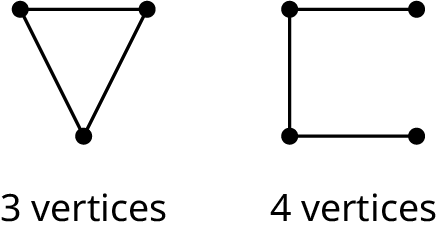Unequal number of edgesUnequal number of vertices of a particular degree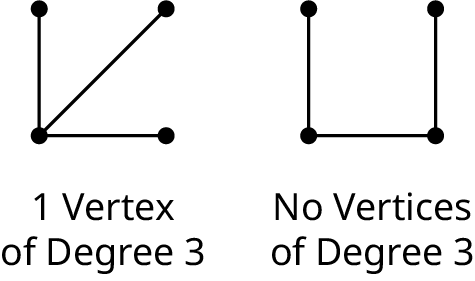Different cyclic subgraphs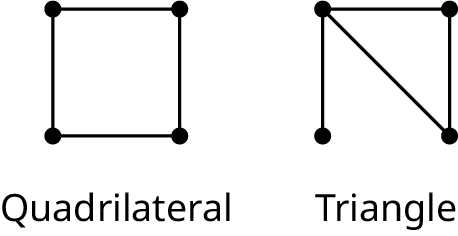Table 12.3 Characteristics of Graphs That Are Not Isomorphic

### Recognizing Isomorphic Graphs

Isomorphic graphs that represent the same pattern of connections can look very different despite having the same underlying structure. The edges can be stretched and twisted. The graph can be rotated or flipped. For example, in Figure 12.41, each of the diagrams represents the same pattern of connections.

Figure 12.41 Four Representations of the Same Graph

Looking at Figure 12.41, how can we know that these graphs are isomorphic? We will start by checking for any obvious differences. Each of the graphs in Figure 12.41 has four vertices and five edges; so, there are no differences there. Next, we will focus on the degrees of the vertices, which have been labeled in Figure 12.42.

Figure 12.42 Graphs with Vertices of the Same Degrees

As shown in Figure 12.42, each graph has two vertices of degree 2 and two vertices of degree 3; so, there are no differences there. Now, let’s check for cyclic subgraphs. These are highlighted in Figure 12.43.

Figure 12.43 Graphs with the Same Cyclic Subgraphs

As shown in Figure 12.43, each graph has two triangles and one quadrilateral; so, no differences there either. It is beginning to look likely that these graphs are isomorphic, but we will have to look further to be sure.

To know with certainty that these graphs are isomorphic, we need to confirm one of the two conditions from the definition of isomorphic graphs. With smaller graphs, you may be able to visualize how to stretch and twist one graph to get the other to see if condition 1 holds. Imagine the edges are stretchy and picture how to pull and twist one graph to form the other. If you can do this without breaking or adding any connections, then the graphs are really the same. Figure 12.44 demonstrates how to change graph A4 to get A3, graph A3 to get A2, and graph A2 to get A1.

Figure 12.44 Transforming Graphs

Now that we have used visual analysis to see that condition 1 holds for graphs A1, A2, A3, and A4 in Figure 12.43, we know that they are isomorphic. In Figure 12.44, one of the edges of graph A4 crossed another edge of the graph. By transforming it into graph A3, we have “untangled” it. Graphs that can be untangled are called planar graphs. The complete graph with five vertices is an example of a nonplanar graph-that means that, no matter how hard you try, you can’t untangle it. But, when you try to figure out if two graphs are the same, it can be helpful to untangle them as much as possible to make the similarities and differences more obvious.

### Example 12.13

#### Identifying Isomorphic Graphs

Which of the three graphs in Figure 12.45 are isomorphic, if any? Justify your answer.

Figure 12.45 Three Similar Graphs

1.
Name four differences between Graph C1 and Graph C2 that show they cannot be isomorphic.
Two Distinct Graphs

Have you ever noticed that many popular board games may look different but are really the same game? A good example is the many variations of the board game Monopoly®, which was submitted to the U.S. Patent Office in 1935. Although the rules have been revised a bit, a very similar game board is still in use today. There have been many versions of Monopoly over the years. Many have been stylized to reflect a popular theme, such as a show or sports team, while retaining the same game board structure. If we were to represent these different versions of the game using a graph, we would find that the graphs are isomorphic. . Let's analyze some game boards using graph theory to determine if they have the same structure despite having different appearances.

Figure 12.48 Two Game Boards

### Example 12.14

#### Deciding If Graphs are Isomorphic

A teacher uses games to teach her students about colors and numbers as shown in Figure 12.48.

In the Colors Game, shown in Figure A, each player begins in the space marked START and proceeds in a counterclockwise direction. On each turn, the player spins a spinner marked 1 and 2 and moves forward the number of spaces shown on the spinner. If the player lands on a space marked with any color other than white, the player must move forward or back to the other space of the same color. The first player to land in or pass the space marked END wins.

In the Clock Game, shown in Figure B, each player begins in the space marked START and proceeds in a clockwise direction. On each turn, the player spins a spinner marked 1 and 2 and moves forward the number of spaces shown on the spinner. In the same turn, the player must read the number in the space and move forward the number of spaces indicated by a positive value or backward the number of spaces indicated by a negative value. Then the turn ends. The first player to land in or pass the space marked END wins.

Draw a graph or multigraph to represent each game in which the vertices are the spaces and the edges represent the ability of a player to move between the spaces either by a spin or as dictated by a marked color or number. (We will ignore the direction of motion for simplicity.) Transform one of the graphs to show that it is isomorphic to the other and explain what this tells you about the games.

1.
Let's compare the games in Figures A and B. In the game Diamonds, shown in Figure A, each player begins in the space marked START and proceeds in a counterclockwise direction. On each turn, the player rolls a six-sided die and moves forward the number of spaces shown on the die. If the player lands on a space marked with a diamond, the player jumps to the next space marked with a diamond and stops there. The first player to land in or pass the space marked END wins. In the Dots game, shown in Figure B, each player begins in the space marked 1 and proceeds in numerical order through the numbered spaces. On each turn, the player rolls a six-sided die and moves forward the number of spaces shown on the die. When a player lands on a space marked with a green dot, they immediately move to the space indicated by the arrow. Construct a graph to represent each gameboard such that each vertex represented a space on the game board and each edge represented the ability of a player to move directly from one space to the next. For example, in the Dots game, the vertex representing space 3 would be adjacent to 2, 4, and 6. Identify at least three differences between a graph based on Diamonds and a graph based on Dots to show the graphs are not isomorphic.
Two Game Boards

### People in Mathematics

#### Elizabeth Magie

Game designer, engineer, comedian, and political activist, Elizabeth Magie designed a game called “Landlord’s Game” to educate fellow citizens about the dangers of monopolies and the benefits of wealth redistribution through a land tax. Magie, whose father had campaigned with Abraham Lincoln, was a proponent of a land tax, an idea popularized by Henry George’s 1879 book, Progress and Poverty. She designed the game to be played by two sets of rules for comparison. In one version, the goal was to dominate opponents by creating monopolies, leaving one wealthy player standing in the end. In the other version, all the players were rewarded when a monopoly was created through a simulated land tax. She patented this game for the first time in 1904. Does the game board in Figure 12.50 look familiar?

Figure 12.50 Landlord’s Game Patent (credit: "Landlord's Game Patent" Wikimedia Commons, Public Domain)

That’s right! A modified version of the game was obtained from friends by a man named Charles Darrow who renamed it Monopoly and sold to Parker Brothers. Parker Brothers later paid Magie \$500 for the right to her patent on it. The property tax version was left behind, and the modern game of Monopoly was born. (Mary Pilon, Monopoly’s Lost Female Inventor, September 1, 2018, National Women’s History Museum, “Monopoly’s Lost Female Inventor,”

### Identifying and Naming Isomorphisms

When two graphs are isomorphic, meaning they have the same structure, there is a correspondence between their vertices, which can be named by listing corresponding pairs of vertices. This list of corresponding pairs of vertices in such a way that any adjacent pair in one graph corresponds to an adjacent pair in the other graph is called an isomorphism. Consider the isomorphic graphs in Figure 12.38. In Figure 12.51, we could replace the labels Graph F with the labels from Graph A and have an identical graph, as in Figure 12.52.

Figure 12.51 Identical Graphs with Different Labels
Figure 12.52 Corresponding Vertices

So, we can identify an isomorphism between Graph A and Graph F by listing the corresponding pairs of vertices: b-g, c-h, d-i, and e-j. Notice that b is adjacent to c and g is adjacent to h. This must be the case since b corresponds to g and c corresponds to h. The same is true for other pairs of adjacent vertices.

An isomorphism between graphs is not necessarily unique. There can be more than one isomorphism between two graphs. We can see how to form a different isomorphism between Graph A and Graph F from Figure 12.33 by rotating Graph F clockwise and comparing the rotated version of F to Graph A as in YOUR TURN 12.13. Now, we can see that a second isomorphism exists, which has the correspondence: b-j, d-h, c-i, and e-g as shown in Figure 12.53.

Figure 12.53 Transforming Graph F into Graph A

### Checkpoint

When you name isomorphisms, one way to check that your answer is reasonable is to make sure that the degrees of corresponding vertices are equal.

### Example 12.15

#### Identifying Isomorphisms

In Example 12.13, we showed that the Graphs B1 and B2 in Figure 12.45 are isomorphic. In Figure 12.54, labels have been assigned to the vertices of Graphs B1 and B2. Identify an isomorphism between them by listing corresponding pairs of vertices.

Figure 12.54 Two Isomorphic Graphs

1.
Graphs E and T are isomorphic. Name two isomorphisms.
Graph E and Graph T

### Example 12.16

#### Recognizing an Isomorphism

Determine whether Graphs G and S in Figure 12.56 are isomorphic. If not, explain how they are different. If so, name the isomorphism.

Figure 12.56 Graph G and Graph S

1.
Three students have been asked to determine if Graphs E and T are isomorphic and justify their answers.
Graph E and Graph T

Javier believes that Graphs E and T are not isomorphic because Graph E contains a triangle cycle and Graph T does not. He supports his conclusion with the graphs shown.
Javier’s Highlighted Cycle
Maubi also believes that Graphs E and T are not isomorphic, but says that it is because Graph T has a quadrilateral cycle (p, q, r, s) while Graph E does not. Caden believes that Graphs E and T are isomorphic. She said one isomorphism is a-p, b-q, c-s, and d-r. Determine who is correct, who is incorrect, and explain how you know.

### Complementary Graphs

Suppose that you are a camp counselor at Camp Woebegone and you are holding a camp Olympics with four events. The campers have signed up for the events. You drew a graph in Figure 12.58 to help you visualize which events have campers in common.

Figure 12.58 Graph of Events with Campers in Common

Graph E in Figure 12.58 shows that some of the same campers will be in events a and b, as well as b and d, c and d, and a and c. What do you think the graph would look like that represented the events that do not have campers in common? It would have the same vertices, but any pair of adjacent edges in Graph E, would not be adjacent in the new graph, and vice versa. This is called a complementary graph, as shown in Figure 12.59. Two graphs are complementary if they have the same set of vertices, but any vertices that are adjacent in one, are not adjacent in the other. In this case, we can say that one graph is the complement of the other.

Figure 12.59 Graphs of Camp Olympics

One way to find the complement of a graph is to draw the complete graph with the same number of vertices and remove all the edges that were in the original graph. Let’s say you wanted to find the complement of Graph E from Figure 12.59, and you didn’t already know it was Graph F. You could start with the complete graph with four vertices and remove the edges that are in Graph E as shown in Figure 12.60.

Figure 12.60 Use Complete Graph to Find Complement

### Example 12.17

#### Finding a Complement

A particular high school has end-of-course exams in (E3) English 3, (E4) English 4, (M) Advanced Math, (C) Calculus, (W) World History, (U) U.S. History, (B) Biology, and (P) Physics. No English 3 students are taking English 4, World History, or Biology; no English 4 students are also in Calculus, Advanced Math, U.S. History, or Physics; no Physics students are also taking Advanced Math; No World History students are also taking U.S. History; and no Advanced Math students are also taking Calculus.

1. Create a graph in which the vertices represent the exams, and an edge between a pair of vertices indicates that there are no students taking both exams.
2. Find the complement of the graph in part 1.
3. Explain what the graph in part 2 represents.

1.
Find the complement of the Graph H.

When two graphs are really the same graph, they have the same missing edges. So, when two graphs have a lot of edges, it may actually be easier to determine if they are isomorphic by looking at which edges are missing rather than which edges are included. In other words, we can determine if two graphs are isomorphic by checking if their complements are isomorphic.

### Example 12.18

#### Using a Complement to Find an Isomorphism

Use Figure 12.64 to answer each question.

Figure 12.64 Graph K
1. Find the complement of Graph K.
2. Identify an isomorphism between the complement of Graph K from part 1, and the complement of Graph H in YOUR TURN 12.17.
3. Confirm that the correspondence between the vertices you found in part 2 also gives an isomorphism between Graph H from YOUR TURN 12.17, and Graph K from Figure 12.64.

1.
Suppose that a Graph M is a complete graph and Graph N is the complement of M. What are the degrees of the vertices of Graph N? How do you know?

### WORK IT OUT

Here is an activity you can do with a few of your classmates that will build your graph comparison skills.

Step 1: Draw a planar graph with the following characteristics: exactly five vertices, one vertex of degree four, at least two vertices of degree three, and exactly eight edges. Give names to the vertices. Make sure you do not use the same letters or numbers to label your vertices as your classmates do.

Step 2: Analyze your graph. What is the degree of each vertex? Does your graph have any cyclic subgraphs? If so, list them and indicate their sizes.

Step 3: Draw and analyze the complement of your graph. How many edges and vertices does it have? What is the degree of each vertex? Does the complementary graph have any cyclic subgraphs? If so, list them and indicate their sizes.

Step 4: Compare your graphs to each of your classmates’ graphs. Does your graph have the same number of edges and vertices as the graph of your classmate? Does your graph have the same size cyclic subgraphs as the graph of your classmate? How does the complement of your graph compare to the complement of the graph of your classmate? Determine if your graph is isomorphic to your classmates’ graph. If so, give a correspondence that demonstrates the isomorphism. If not, explain how you know.

For the following exercises, determine whether each statement is always true, sometimes true, or never true.
14.
Two graphs are isomorphic, and the graphs have the same structure.
15.
A graph with four vertices is isomorphic to a graph with five vertices.
16.
The sums of the degrees of the vertices of two graphs are equal, but the two graphs are not isomorphic.
17.
Two graphs are isomorphic, but the graphs have a different number of edges.
18.
One graph can be transformed to look like a second graph without removing or adding any connections, and the two graphs are isomorphic.
19.
Two graphs are isomorphic, and there is more than one isomorphism between the two graphs.
20.
Two graphs have the same number of vertices, but there is no isomorphism between them.
21.
There is a correspondence between the vertices of Graph A and the vertices of Graph B such that the adjacent vertices in Graph A always correspond to vertices of Graph B, but the two graphs are not isomorphic
22.
Two graphs have the same number of edges, the same number of vertices, vertices of the same degree, and have all the same subgraphs, but they are not isomorphic
23.
Two graphs are isomorphic, and the sum of the degrees of the vertices of one equals the sum of the degrees of the other graph.
24.
If two graphs are isomorphic, then their complements are isomorphic.

### Section 12.3 Exercises

Use the figure to answer the following exercises. A pair of graphs is given. Identify three differences between them that demonstrate the graphs are not isomorphic.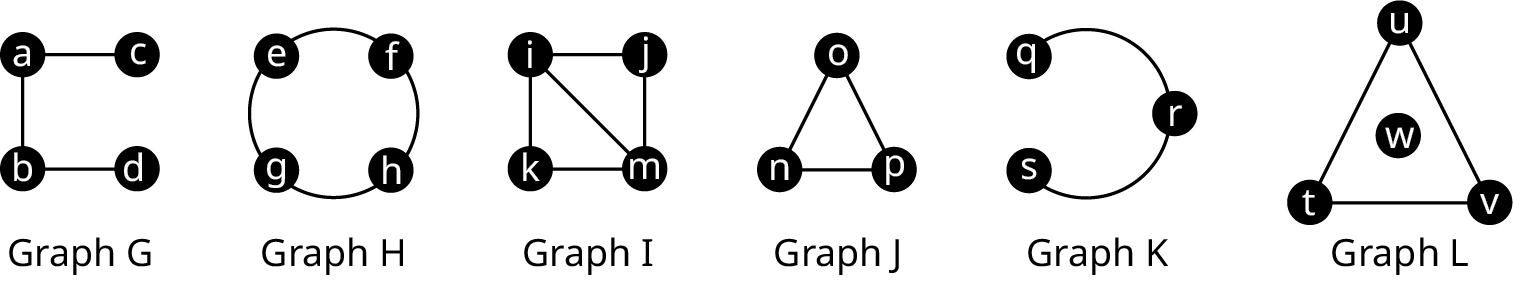1 .
G and H
2 .
G and I
3 .
G and J
4 .
G and K
5 .
G and L
6 .
H and I
7 .
H and J
8 .
H and K
9 .
H and L
10 .
I and J
11 .
I and K
12 .
I and L
13 .
J and K
14 .
K and L
Use the figure to answer the following exercises. In each exercise, a pair of graphs is given. Determine if one graph is a subgraph of the other graph, or the two graphs are isomorphic.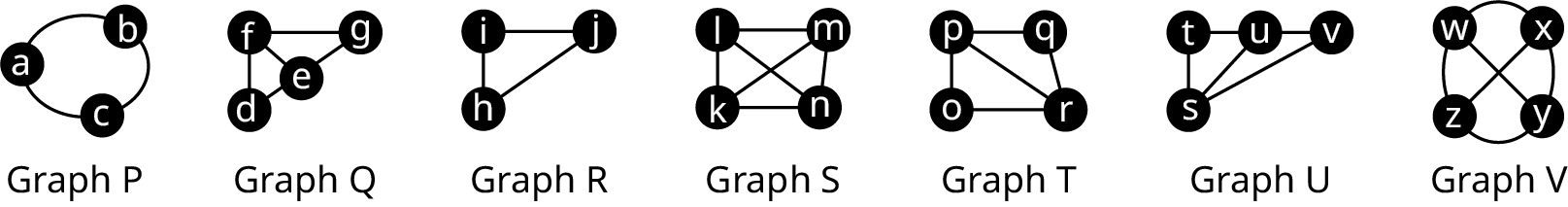15 .
P and Q
16 .
P and R
17 .
P and U
18 .
Q and R
19 .
Q and S
20 .
Q and T
21 .
Q and V
22 .
S and V
23 .
T and U
24 .
U and V
Use the figure to answer the following exercises. In each exercise, a pair of graphs is given. Either give a reason that the graphs are not isomorphic, or show how one of the graphs can be transformed to look like the other.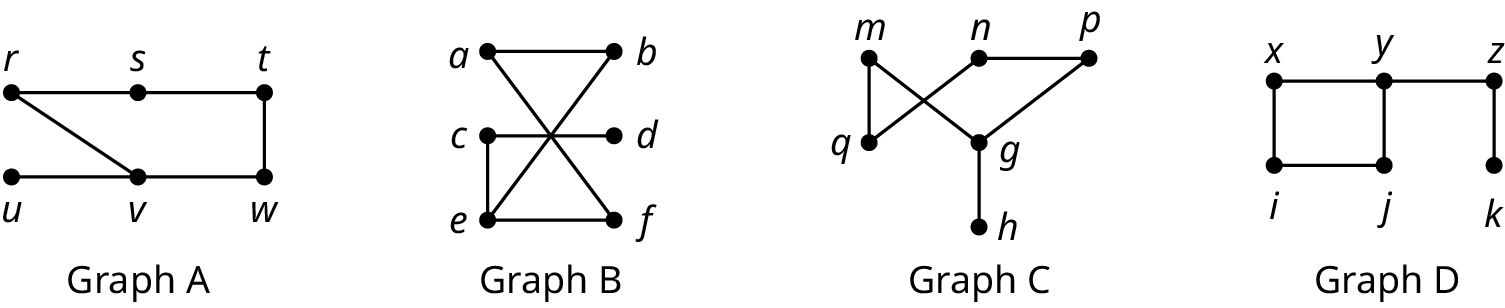25 .
A and B
26 .
A and C
27 .
A and D
28 .
B and C
29 .
B and D
30 .
C and D
Use the figure to answer the following exercises. Determine if one graph is a subgraph of the other graph, or the two graphs are isomorphic. If they are isomorphic, name an isomorphism. If one is a subgraph of the other, indicate a correspondence between the vertices that demonstrates the relationship.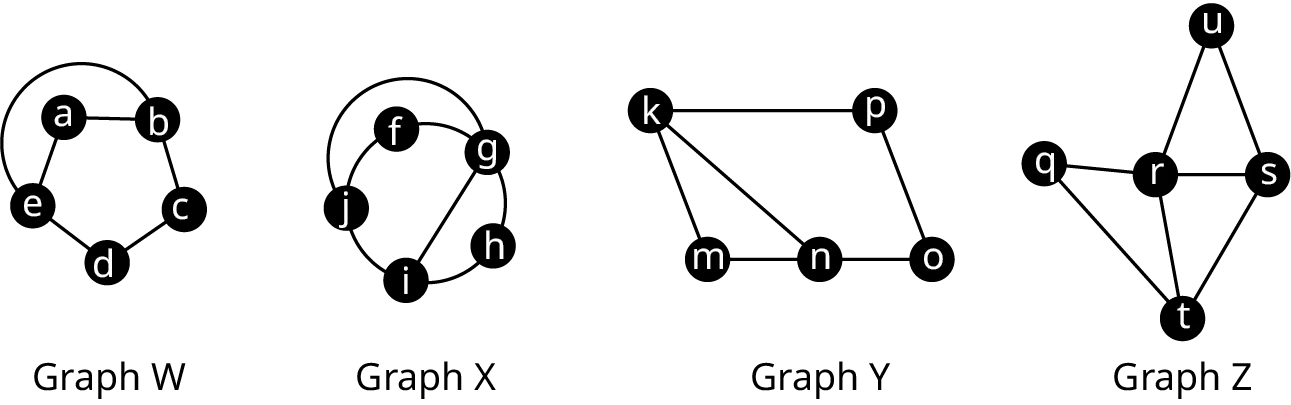31 .
W and Z
32 .
X and Y
33 .
X and Z
Use the figure to answer the following exercises.34 .
Find the complement of Graph W.
35 .
Find the complement of Graph Y.
36 .
Find the complement of Graph X.
37 .
Find an isomorphism between the complement of W and the complement of Y if one exists. If not, explain how you know.
38 .
Are W and Y isomorphic? Explain how you know.
39 .
Find an isomorphism between the complement of W and the Complement of X if one Exists. If not, explain how you know.
40 .
Are W and X isomorphic? Explain how you know.
41 .
Find the complement of the graph in the given figure representing direct flights between south Florida airports. Explain what the graph represents.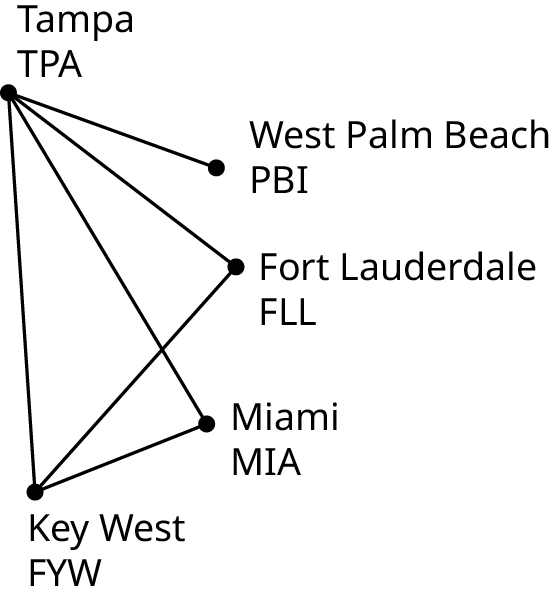Order a print copy

As an Amazon Associate we earn from qualifying purchases.